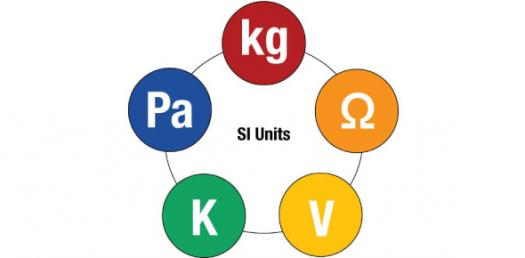Can You Identify The SI Unit? Trivia Quiz

10 Questions | Total Attempts: 89Settings.

• 1.
The SI unit for electromotive force is in ........
• A.

Ampere

• B.

Coulomb

• C.

Volts

• D.

Joules

• 2.
The SI unit for current is in ........
• A.

Ampere

• B.

Coulomb

• C.

Volts

• D.

Joules

• 3.
The SI unit for charge is in ........
• A.

Ampere

• B.

Coulomb

• C.

Volts

• D.

Joules

• 4.
The SI unit for electromotive force is in ........
• A.

A

• B.

C

• C.

V

• D.

J

• 5.
The SI unit for potential difference is in ........
• A.

Ampere

• B.

Coulomb

• C.

Volts

• D.

Joules

• 6.
The SI unit for energy is in ........
• A.

Ampere

• B.

Coulomb

• C.

Volts

• D.

Joules

• 7.
The SI unit for energy is in ........
• A.

A

• B.

C

• C.

V

• D.

J

• 8.
The SI unit for potential difference is in ........
• A.

A

• B.

C

• C.

V

• D.

J

• 9.
The SI unit for charge is in ........
• A.

A

• B.

C

• C.

V

• D.

J

• 10.
The SI unit for current is in ........
• A.

A

• B.

C

• C.

V

• D.

J

Related TopicsBack to top